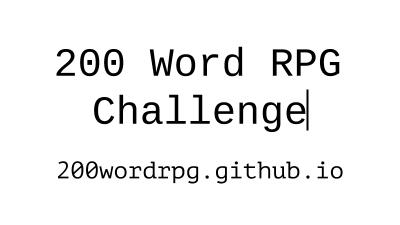Coin flip to dice roll conversion • 2016 supplement

``````A die to coin conversion for traditional D20 system rolls. Useful where dice are scarce.

Coin flips recorded in order can produce random binary numbers of large sizes. Heads are recorded as 1, tails as 0. (ex. 0-1-0-0 = 4, 1-1-0-1 = 13) Brush up on binary, or use a conversion calculator.

Some of these are easy, like the D4. Adding 1 helps to make sure there are no rolls of zero.
D4 = 2 bits +1  (bits = coin flips)
D8 = 3 bits +1
D16(?) = 4 bits +1
D32(?)= 5 bits +1

Numbers that are not divisible by 2^x are more difficult to find. The easiest way to find them is to find the next largest bit increment that contains a number, and discard rolls above it.

D6 = 3 bits, ignored if above (110),

D20 = 5 bits, ignored if its above (10100),

D100 = 7 bits, Ignored if above (1100100)

A few example rolls:

I make a D20 Attack roll. I get (11101) = 29. That’s too high, and must be discarded.
Rolling again, I get (01011) = 11. I go ahead and add my regular bonuses, and use the roll.

I cast a 3d4 magic missile. Damage  = (01)  + (10) + (11) + 3  = 9 + Usual bonuses.
``````